Importance: High ✭✭✭
 Author(s): Graham, Ronald L.
 Subject: Graph Theory
 Keywords: pebbling zero sum
 Posted by: mdevos on: September 24th, 2007

We let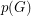denote the pebbling number of a graph.

Conjecture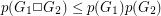.

The pebbling number of a graph, denoted, is the smallest integerso that howeverpebbles are distributed onto the vertices of, it is possible to move a pebble to any vertex by a sequence of steps, where in each step we remove two pebbles from one vertex, and then place one on an adjacent vertex. The cartesian product of two graphs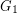and, denoted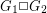, is the graph with vertex set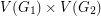and an edge fromto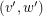if either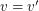and(in) or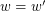and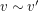(in).

Graph Pebbling arose out of the study of zero-sum subsequences in groups, but has proved to be a rich and interesting topic in graph theory. See Glenn Hurlbert's wonderful graph pebbling page for much more on this topic (and this problem in particular). Graham's conjecture was stated in one of the first papers on this subject by Fan Chung [C], and has since generated considerable interest.

There are a number of partial results toward this conjecture. Chung [C] proved that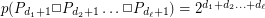, thus settling the conjecture for products of paths (heredenotes a path withvertices). It is also known whenare both trees, both cycles, and for graphs with high minimum degree. Again, we encourage the interested reader to see the graph pebbling page for more details.

## Bibliography

*[C] F. Chung, Pebbling in hypercubes SIAM J. Disc. Math. 2 (1989), 467--472.

* indicates original appearance(s) of problem.